×#### Thank you for registering.

One of our academic counsellors will contact you within 1 working day.

Click to Chat

1800-1023-196

+91-120-4616500

CART 0

• 0

MY CART (5)

Use Coupon: CART20 and get 20% off on all online Study Material

ITEM
DETAILS
MRP
DISCOUNT
FINAL PRICE
Total Price: Rs.

There are no items in this cart.
Continue Shopping```Monotonocity

Table of Content

Derivative as a Rate of Change

Monotonocity

Increasing Function

Non-Decreasing Function

Decreasing Function

Non-Increasing Function

Derivative as a Rate of ChangeLet s be the displacement described by a particle in time t. Then s will be a function of t and we will write, s = f(t) units.

The velocity 'v' of the particle at time t is, v = ds/dt = f'(t) units.

The acceleration 'f' at time t, that is, the rate of change of velocity at time t is; f = dv/dt=d2s/dt2 = f"(t) units.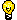Note: Here we are considering only magnitude of displacement, velocity and acceleration.

Illustration:

A body of mass 6g is in rectilinear motion according to the law,

s = - 1 + ln (t + 1) + (t + 1)3, (s is in centimeters and t, in seconds). Find the kinetic energy (1/2 mv2 ) of the body one-second after it begins to move.

Solution:

The speed of the body is equal to the time-derivative of the distance:

v(t) = ds/dt=1/(t+1) + 3(t + 1)2.,    v(l) = 12 1/2 cm/sec.

Kinetic energy = 1/2 mv2 = 1/2 (6) (25/2)2 = 468 3/4 (erg.)

Monotonocity

A function f(x) is said to have a monotonic behavior if it is either non increasing or non decreasing in a given domain. In statement form, it can be stated as:

If y = f(x) represent a non decreasing function in [a, b] then for every x1 > x2; x1, x2 Î [a, b] we have y1 > y2 or equivalently we can say that dy/dx > 0 in (a, b)

If we have strict inequality sign, the function is said to be strictly increasing (or strictly monotonic).

Similarly if f(x) is a non increasing function in [a, b] we have ∀ x1 > x2; x1, x2 Î [a, b] Þ f(x1) < f(x2) or equivalently, f'(x) < 0.

If we have strict inequality sign the function is said to be strictly decreasing (or strict monotonic). Any function increasing/decreasing is said to be strictly monotonic if f'(x) > 0 or f'(x) < 0

Let y = f(x) be a given function with 'D' as it's domain. Let D1 ⊆ D.

Increasing Function

q  f(x) is said to be increasing in D1 if for every x1, x2 ∈ D1, x1 > x2

=>  f(x1) > f(x2).

It means that the value of f(x) will keep on increasing with an increase in the value of x. Refer to  the Figure given below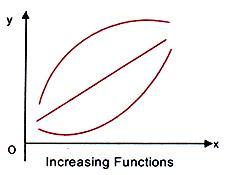Non-Decreasing Function

q  f(x) is said to be non-decreasing in D1 if for every x1, x2 ∈ D1, x1 > x2

=>  f(x1) > f(x2).

It means that the value of f(x) would never decrease with an increase in the value of x. Refer to the Figure given below.Decreasing Function

q  f(x) is said to be decreasing in D1 if for every x1, x2 ∈ D1, x1 > x2.

=>     f(x1) < f(x2).

It means that the value of f(x) would increase with an increase in the value of x. Refer to the Figure given below.Non-Increasing Function

q  f(x) is said to be non-increasing in D1 if for every x1, x2 c D1, x1 > x2.

=> f(x1) < f(x2).

It means that the value of f(x) would never increase with an increase in the value of x. Refer to the Figure given below.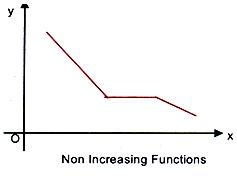Differentiating the equation of a curve and analyzing the behavior of the differential coefficient, one can infer the range in which the curve has as increasing behavior w.r.t. increasing independent variable or curve has a decreasing behaviour w.r.t. increasing independent variable. For example, if we look at the Figure given below,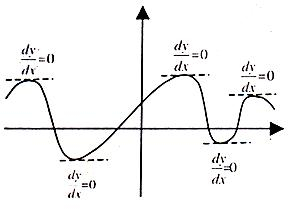It can be seen that when the curve is increasing, dy/dx is positive and when the curve is decreasing, dy/dx is negative (all discussion is based for increasing x). So when the curve changes its behavior i.e. from increasing to decreasing or vice versa, then dy/dx in figure changes from positive to negative or vice versa. So at any intermediate point dy/dx = 0. One can find the range in which curve has either increasing or decreasing behavior by solving the equation  dy/dx = 0.

Illustration:

Let f(x) = x4/4-8x3/3+(19x^2)/2 - 12x + 6. Find the intervals of monotonocity of f(x).

f'(x) = x3 - 8x2 + 19x - 12

= x3 - 8x2 + 8x + 12x - 12 - x

= (x3 - x) + (8x) (1 - x) + 12(x - 1)

= x(x + 1)(x - 1) - 8x(x - 1) + 12 (x - 1)

= (x - 1)(x2 + x - 8x + 12)

= (x - 1)(x2 - 7x + 12)

= (x - 1)(x - 3)(x - 4)

Now, f'(x) = 0 => = 1, 3, 4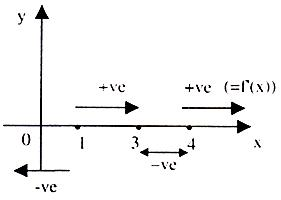These three values of x divide x-axis into four intervals.

For any x c (4, a), f'(x) > 0

Similarly for other interval, the sign of f'(x) is shown in the figure.

f(x) increasing ∀ x ∈ (1, 3) U (4, µ)

f(x) is decreasing ∀ x ∈ (- µ, +1) u (3, 4)

Basic Theorems:

Let, f(x) be a function that is continuous in [a, b] and differentiable in (a, b). Then

(i) f(x) is a non-decreasing function in [a, b] if f'(x) > 0 in (a, b);

(ii) f(x) is an increasing function in [a, b] if f'(x) > 0 in (a, b);

(iii) f(x) is a non-increasing function in [a, b] if f'(x) < 0 in (a, b);

(iv) f(x) is a decreasing function in [a, b] if f'(x) < 0 in (a, b).

Remarks:

(i)  If f'(x) > 0 ∀ x ∈ (a, b) and points which make f'(x) equal to zero (in between (a, b)) don't form an interval, then f(x) would be increasing in [a, b].

(ii)  If f'(x) < 0 ∀ x ∈ (a, b) and points which make f'(x) equal to zero (in between (a, b)) don't form an interval, f(x) would be decreasing in
[a, b]

(iii)  If f(0) = 0 and f'(x) > 0 ∀ x ∈ R, then f(x) < 0 ∀ x ∈ (-∞, 0) and

f(x) > 0 ∀ x ∈ (0, ∞)

(iv)  If f(0) = 0 and f'(x) < 0 ∀ x ∈ R, then f(x) > 0 ∀ x ∈ (-∞, 0) and

f(x) < 0 ∀ x ∈ (0, ∞)

(v)  A function is said to be monotonic if it's either increasing or decreasing.

(vi) The points for which f'(x) is equal to zero or doesn't exist are called critical points. Here it should also be noted that critical points are the interior points of an interval.

(vii)  The stationary points are the points where f'(x) = 0 in the domain.

Illustration:

Prove that sinx + tanx > 2x, when 0 < x < p/2.

Solution:

Let f(x) = sin x + tan x - 2x, x ∈ (0, p/2)

=> f'(x) = cos x + sec2 x - 2.

As cos x > cos2x, x ∈ (0, p/2),

f'(x) > cos2 x + sec2 x - 2 = (cos x - sec x)2 > 0, x ∈ (0, p/2)

Hence f(x) is increasing in (0, p/2).

Since f(x) > lim(x-->0) f(x), for all x ∈ (0, p/2),

f(x) > f(0) => sinx + tanx > 2x.

To read more, Buy study materials of Applications of Derivatives comprising study notes, revision notes, video lectures, previous year solved questions etc. Also browse for more study materials on Mathematics here.
```### Course Features

• 731 Video Lectures
• Revision Notes
• Previous Year Papers
• Mind Map
• Study Planner
• NCERT Solutions
• Discussion Forum
• Test paper with Video Solution